# Vector worked examples

Example 1

Write in terms of a  and b  the vector vec (BC)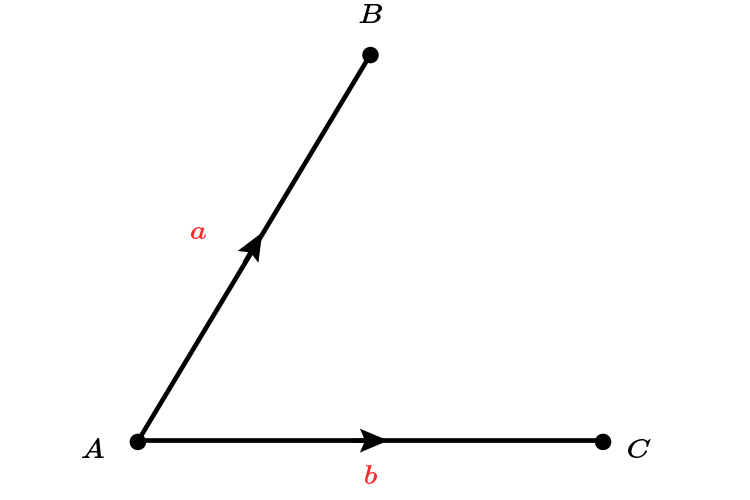Think of this as two separate vectors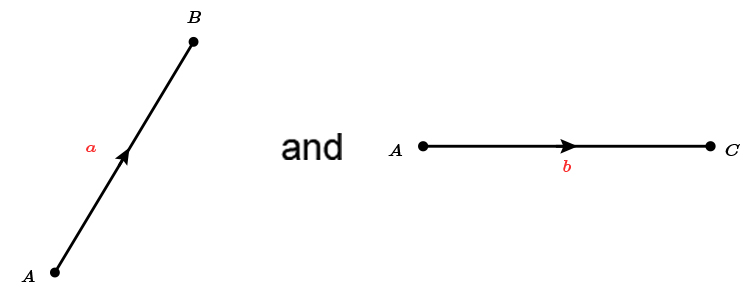Therefore

vec (BC)=-a+b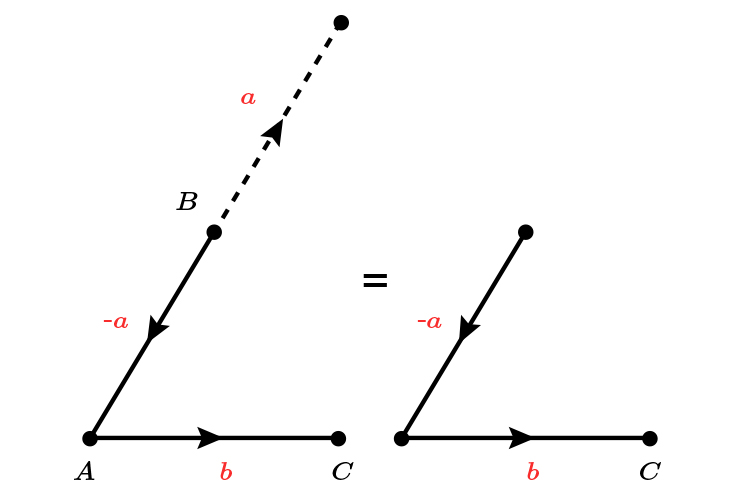Or vec (BC)=b-a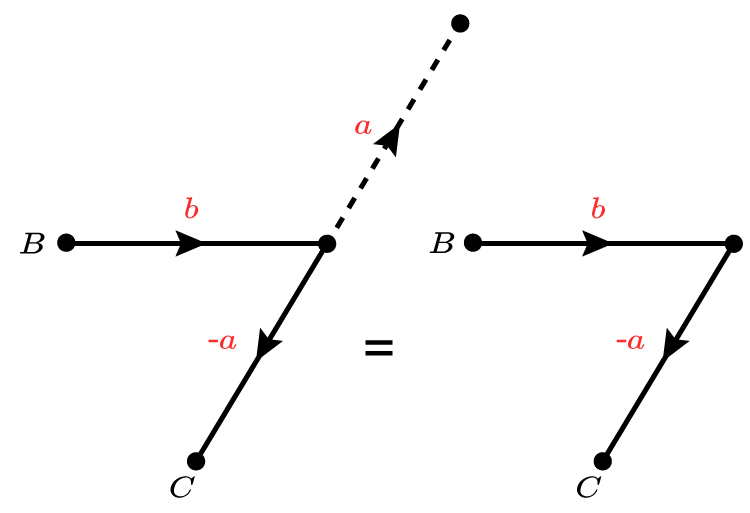Example 2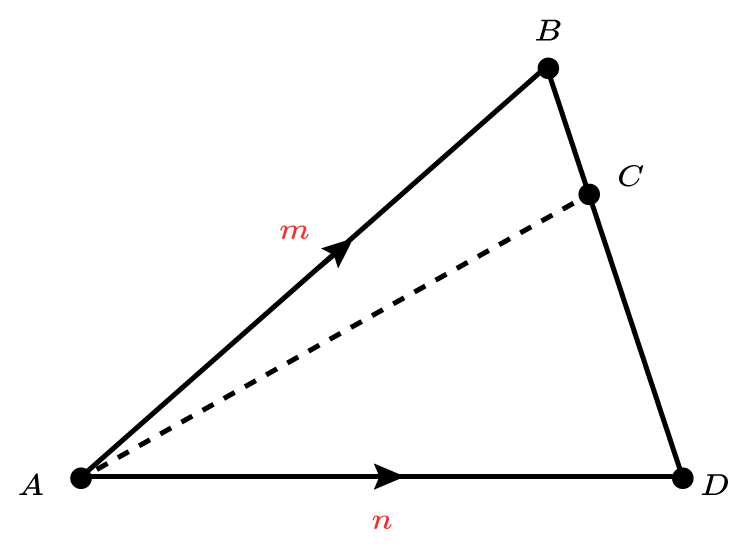vec (AB)=m

vec (AD)=n

The point C  on BD  is such that:

BC:CD=1:3

i.  Find D  to B  in terms of n  and m

Think of this as the separate vectors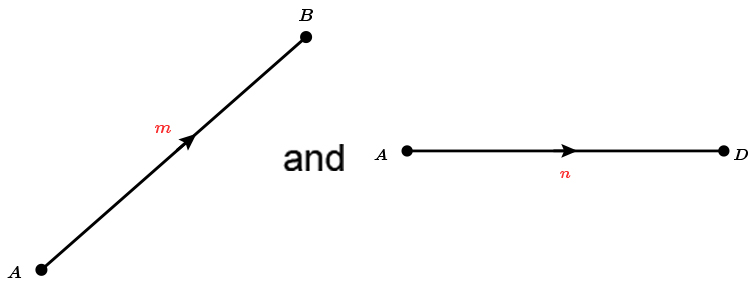Therefore

vec (DB)=-n+m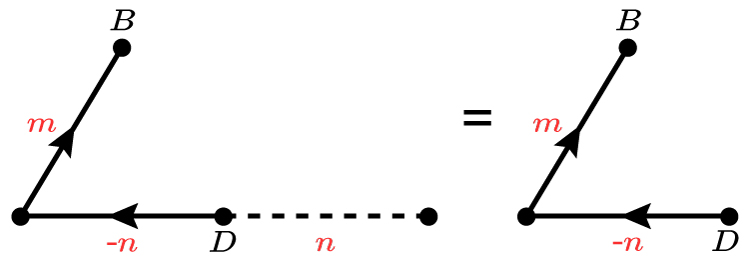Or vec (DB)=m-n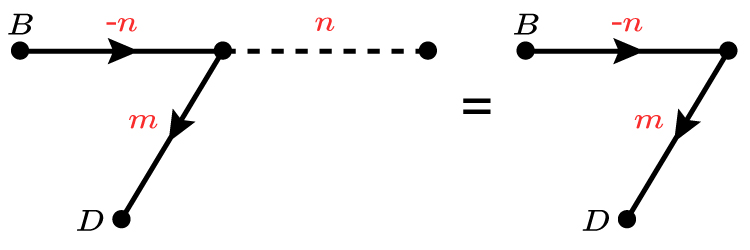vec (DB)=-n+m=m-n   (The latter is chosen because it's neater)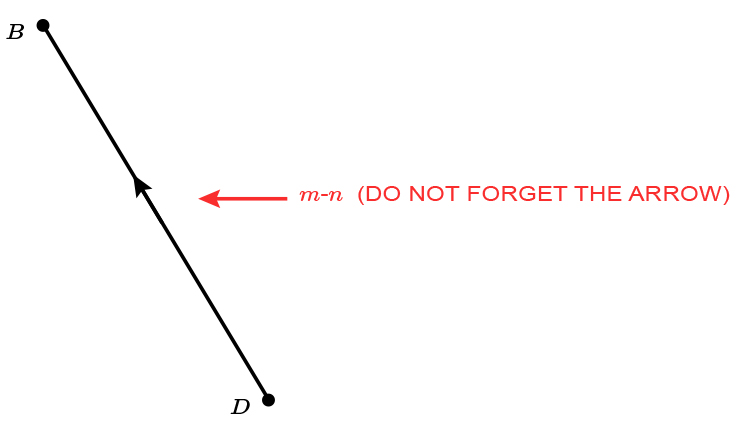ii. Find vec (AC)  in terms of m  and n

remember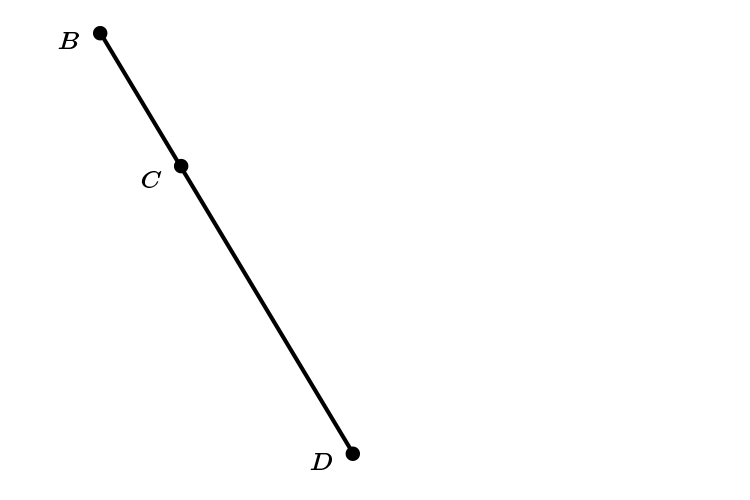We are told BC:CD=1:3

this means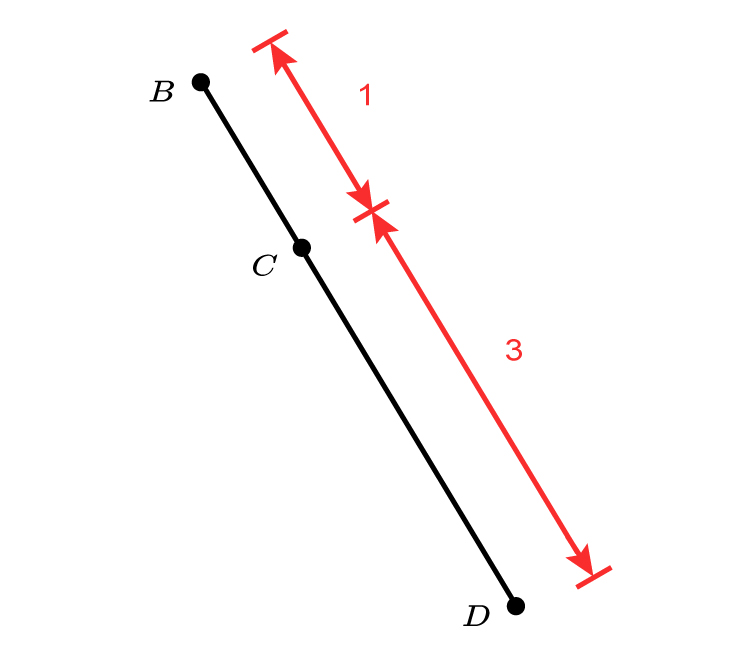which also means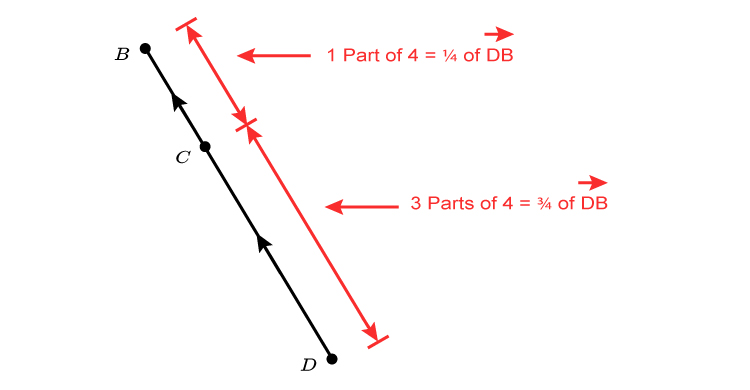which also means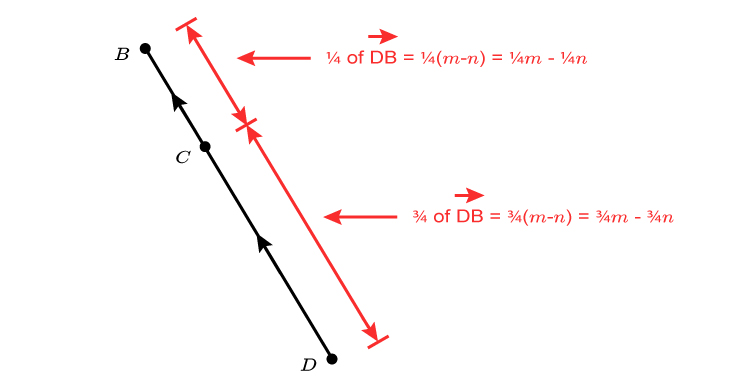So if we redraw the original diagram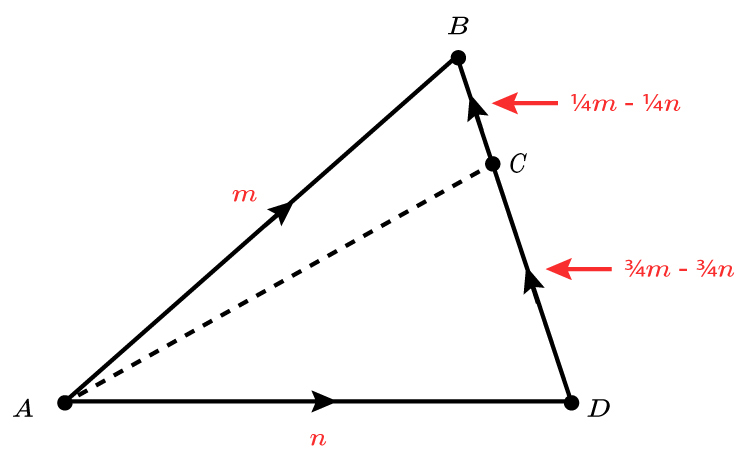vec (AC)  in terms of m  and n  are:

vec (AC)=n+3/4m-3/4n

vec (AC)=3/4m+1/4n

OR

vec (AC)=m-(1/4m-1/4n)

vec (AC)=m+(-1times(1/4m-1/4n))

vec (AC)=m-1/4m+1/4n

vec (AC)=3/4m+1/4n

Both get the same answer (as they should)

vec (AC)  in terms of m  and n=3/4m+1/4n

Example 3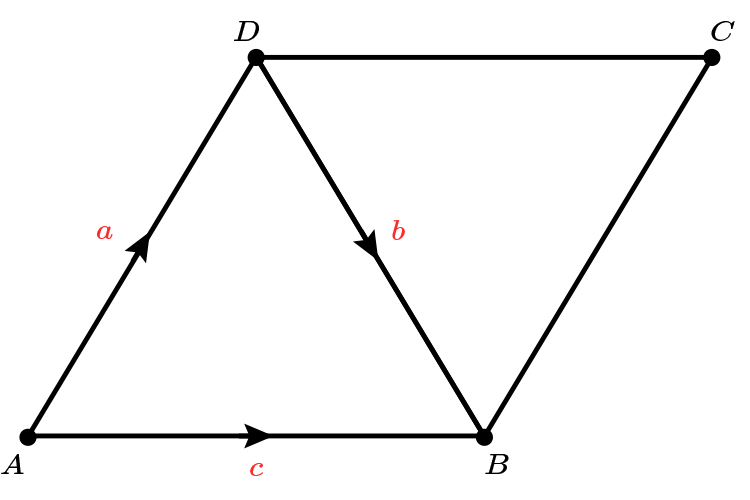If AD  is parallel to CB  and AB  is parallel to DC, write in terms of a  and b  the vectors vec (BC)  and vec (BA)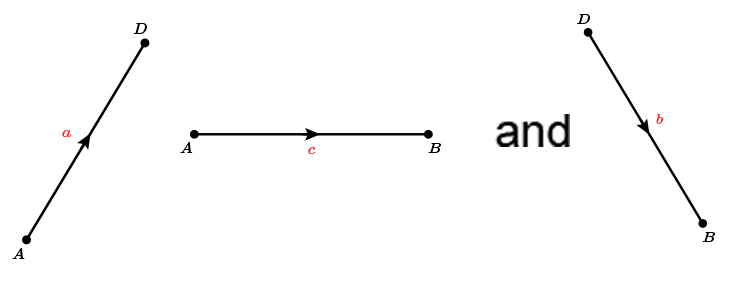vec (BC)=a
vec (BA)=-c